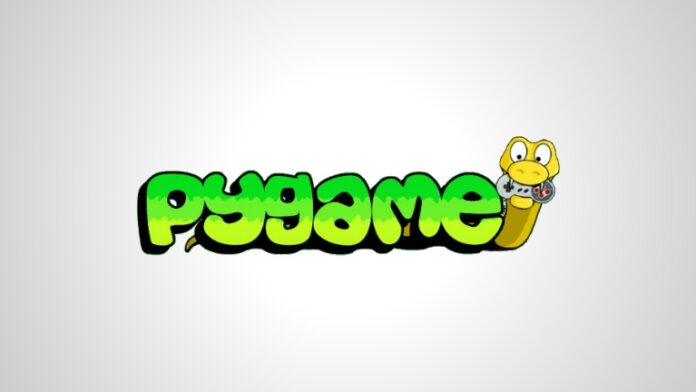# An Introduction To PyGame: A Game In PythonHello there! We are back with another useful and interesting article for you. Yes, this time we are going to tell you how you can make a game in Python with an introduction to PyGame.

While we have previously given many introducing articles for different things, none can be as fun as this. Why, of course, who doesn’t like games?

And why use Python for that? Because it’s one of the most beginner-friendly programming languages you can ask for.

But since it’s for beginners, let’s start at the beginning- the introduction to PyGame.

### Introduction to PyGame- what is it?

Any programming language has its own “software development kit”. You have to know not just the language, but also the tools that come with this kit.

You may already have learned basic Python or a little more than the basics, but that is not enough. To really start developing a game or any other app, you have to be aware of some tools.

To start building something using Python, you need “modules”. Modules are the additional tools that other developers will provide you- they are building blocks.

Different modules perform different functions that you need in order to develop a game or any other app. PyGame is a similar module which supplies a set of different functions which will be useful in the development of a game.

PyGame will provide you with many ready-made functions like graphics and playing sound. This will, therefore, save you a lot of extra hard work and time.

### Introduction to PyGame- first step

Before we start here, you must have some basic knowledge of Python. Those who are not aware of the basics must do so- take a tutorial.

So assuming that you know the basics, let’s move forward. First things first- you need a Python IDE or code editor to start with.

Here is a code which we will go through step by step soon:

```import pygame
pygame.init()
win = pygame.display.set_mode((1280, 720))
pygame.display.set_caption("Squarey")
x = 100
y = 100
vel = 6
run = True
def drawGame():
win.fill((0, 0, 0))
pygame.draw.rect(win, (0, 0, 255), (x, y, 20, 20))
pygame.display.update()

while run:
pygame.time.delay(100)

drawGame()
else:
run = False

for event in pygame.event.get():
if event.type == pygame.QUIT:
run = False

keys = pygame.key.get_pressed()

if keys[pygame.K_LEFT]:
x -= vel

if keys[pygame.K_RIGHT]:
x += vel

if keys[pygame.K_UP]:
y -= vel

if keys[pygame.K_DOWN]:
y += vel

drawGame()

pygame.quit()```

Now that you have seen this code, let’s break it down and see how this works.

### How does this work?

Each code has its own importance. First of all, we will import the game by line import pygame. The next step is to initialize PyGame by typing pygame.init().

Now we open the window which displays our game. “Set_caption” lets you set a name for your game.

```import pygame
pygame.init()
win = pygame.display.set_mode((1280, 720))
pygame.display.set_caption("Squarey")```
```Now we have to define a few variables, coordinates and booleans which can tell us if the game is running or not. This is done in this part:
x = 100
y = 100
vel = 6
run = True
Now moving on to next step, have a look at following part of the code.
def drawGame():
win.fill((0, 0, 0))
pygame.draw.rect(win, (0, 0, 255), (x, y, 20, 20))
pygame.display.update()```

The next function is called drawGame(). In this function, we will fill the screen with a blank colur which will help characters move without a trail.

Now we will draw two squares and place them inside the window and give them RGB color codes. Next, we set X and Y coordinates following it up with the addition of width and height.
Now, in the end, pygame.display.update() will get all these elements drawn on the screen.

### Creating a game loop in Python

This part is the “boilerplate”, a loop which will keep repeating if the value of run is set to true. Take a look at the following piece of code. The first line in the code will help in delaying the repetition.

```while run:
pygame.time.delay(100)
drawGame()
else:
run = False```

Now use of if and elif will help in controlling the flow of the code. In case coordinates fall within 10 pixels of the player, the game then the game will be over.